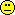## PE vs GMR in Bear [🇷 for BE/BA]

Dear roman_max,

❝ Very often I face with that in BE study reports submitted to EMA/FDA by different companies GMR is the metric, not PE (along with its CIs). This is the reason why I interested in what we really "calculate" and why, is it specific of particular software or using of different terminology or something else.

Yes, the terminology is confusing.
Even if you look into EMA guideline:
The assessment of bioequivalence is based upon 90% confidence intervals for the ratio of the population geometric means (test/reference) for the parameters under consideration.
<...>
The geometric mean ratio (GMR) should lie within the conventional acceptance range 80.00-125.00%.

They are using 'geometric means', 'geometric mean ratio' terms, but adjusted means (aka LSMeans) should be taken into account instead. As an example please see the code and conclusions in EMA PKWP Q&A

❝ In a BE study report there is a summary table for presenting BE results, where geometric means (or LSMEANs?) in natural scale, GMR (%) (or PE(%)?) and CI(%) should be reported.

Common practice is to present GeoLSMeans (backtransformed LSMeans) and their ratio (PE).

❝ MEAN-ref = 3.970504

❝ MEAN-test = 4.097524

❝ MSE = 0.1563787

❝ SE = 0.08338823

❝ Estimate(test-ref) = 0.1282001

4.097524 - 3.970504 = 0.12702❝ Point Estimate CI90 lower CI90 upper

113.678 98.809 130.785

log(113.678) = 0.1281997 = Estimate(test-ref)

❝ As Yung-jin explained me that PE in R/bear is not a direct arithmetic difference, and it is estimated in a specific linear model in ANOVA.

Sounds strange to me. Do we have the ratio of LSMeans, the ratio of GeoMeans and something else?❝ << PK Parameter Summaries >>

❝ summary Test Ref Ratio

❝ LSMEAN 4.097 3.971 1.043

See above:
MEAN-test = 4.097524 which could be rounded to 4.098, not 4.97❝ So, Exp(LSMEAN[T])=60.159 and Exp(LSMEAN[R])=53.038, and these are geometric means, right?

These are GeoLSMeans❝ Does my understanding correct now?

I think we need to wait for Yung-jin. I'm too lazy to dig into BEAR❝ But what to report to authorities? PE or GMR? For balanced study they are equal, but for imbalanced they are slightly different.

GeoLSMs and their ratio is a common practice.
You can try to add a footnote and describe somewhere why GeoMeans could be unequal to LSMeans

❝ And what about CIs? Should I construct them for GMR as well?

See above. Do not try to complicate your lifeKind regards,
MittyriIng. Helmut Schütz